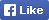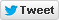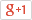Computer/Electronics Questions and Answers
C, C++, VC++, COM/DCOM, DLL and moreLoginSign upHome Electronics Computer Questions Member's Area News Site Map About

Write a program to find the HCF and LCM of the given numbers using functions

Source Code

 1 #include 2 #include 3 int fhcf(int a,int b) 4 { 5 int l,i,hcf; 6 if(a>b) 7 l=a; 8 else 9 l=b; 10 for(i=2;i<=l;i++) 11 { 12 if(a%i==0 && b%i==0) 13 { 14 hcf=i; 15 } 16 } 17 return hcf; 18 } 19 int flcm(int a,int b) 20 { 21 int p=0,i,lcm; 22 p=a*b; 23 for(i=1;i<=p;i++) 24 { 25 if(i%a==0 && i%b==0) 26 { 27 lcm=i; 28 break; 29 } 30 } 31 return lcm; 32 } 33 int main (int argc, char *argv[]) 34 { 35 clrscr(); 36 int a,b,hcf,lcm; 37 printf("\nEnter two numbers:"); 38 scanf("%d %d",&a,&b); 39 hcf=fhcf(a,b); 40 lcm=flcm(a,b); 41 printf("\nHCF of %d and %d is: %d",a,b,hcf); 42 printf("\nLCM of %d and %d is: %d",a,b,lcm); 43 getch(); 44 }

Find More from our code collection
Armstrong number, binary number to a decimal number, bubble sort, decimal number to binary number, factorial of the given number factors, fibonacci numbers, HCF and LCM, matrix, mergesort, salary of the employee. palindrome, quadratic equation, star patterns, series etc. and much more...Return to Example Source Code